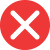simplier

Incorrect spelling, explanation: this word is incorrect because the base adjective simple ends with e, not y (so we don’t add i). The mistake may come from a similar word simply, but it is an adverb, while we need an adjective. Therefore simplier is incorrect. The correct spelling is simpler (simple + -er is simpler).simpler

Correct spelling, explanation: simpler is the correct spelling because the base adjective of this comparative is simple. To create a comparative we add -er or only -r (in case of a word already ending with e, just like here). Therefore simple + -er is simpler. Simplier must be the wrong form then.

Definition of simpler:
adjective, comparative form from simple, less difficult/complicated, more simple
This book is too complicated for me, I prefer simpler stories.
Is this task simpler than the last one?